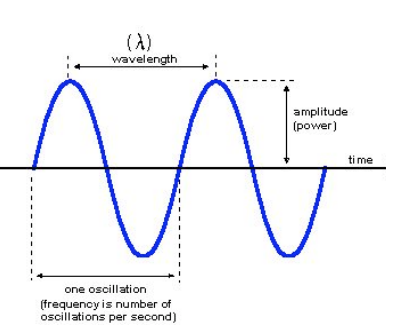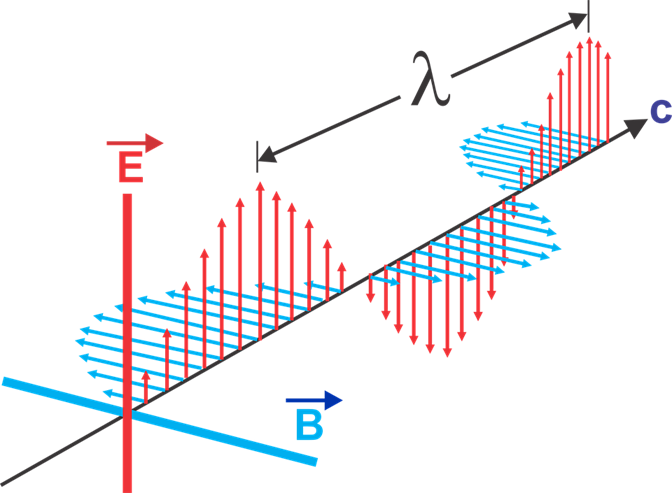# Wave

A wave is a disturbance in a medium that carries energy without a net movement of particles. It may take the form of elastic deformation, a variation of pressure, electric or magnetic intensity, electric potential, or temperature.

## Introduction of Waves

• Transfers energy.
• Usually involves a periodic, repetitive Movement.
• Does not result in a net movement of the medium or particles in the medium (mechanical wave).There are some basic descriptors of a wave. Wavelength is the distance between an identical part of the wave. Amplitude is maximum displacement from the neutral position. This represents the energy of the wave. Greater amplitude carries greater energy. Displacement is the position of a particular point in the medium as it moves as the wave passes. Maximum displacement is the amplitude of the wave

Frequency (ƒ) is the number of repetitions per second in Hz, s -1 Period (T) is the time for one wavelength to pass a point. T = ƒ-1

The velocity (v) of the wave is the speed that a specific part of the wave passes a point. The speed of a light wave is c.

## Types of Waves:

the types of waves are given below.

### Transverse Waves

Waves in which the medium moves at right angles to the direction of the wave.

Examples of transverse waves:

• Water waves (ripples of gravity waves, not sound through water)
• Light waves
• S-wave earthquake waves
• Stringed instruments
• Torsion wave

The high point of a transverse wave is a crest. The low part is a trough.

### Longitudinal Wave:

A longitudinal wave has the movement of the particles in the medium in the same dimension as the direction of movement of the wave.

Examples of longitudinal waves:

• Sound waves
• P-type earthquake waves
• Compression wave

Parts of longitudinal waves:

Compression: where the particles are close together.

Rarefaction: where the particles are spread apart.

### Electromagnetic Waves:

Radio signals, light rays, x-rays, and cosmic rays.

### Mechanical waves:

A wave which needs a medium in order to propagate itself. Sound waves, waves in a Slinky, and water waves are all examples of this.

### Matter Waves:

Any moving object can be described as a wave When a stone is dropped into a pond, the water is disturbed from its equilibrium positions as the wave passes; it returns to its equilibrium position after the wave has passed.

### Electromagnetic Waves:

These waves are the disturbance that does not need any object medium for propagation and can easily travel through the vacuum. They are produced due to various magnetic and electric fields. The periodic changes that take place in magnetic electric fields and therefore known as Electromagnetic WaveElectromagnetic wave

### Wave Speed Formula

It is the total distance covered by the wave in a given time period. The formula for wave speed is given as,

Wave Speed = Distance Covered/Time taken

## Properties of Waves

The prime properties of waves are as follows:

Amplitude – Wave is an energy transport phenomenon. Amplitude is the height of the wave, usually measured in meters. Amplitude is directly related to the amount of energy carried by a wave.

Wavelength – The distance between identical points in the adjacent cycles or crests of a wave is called wavelength. It is also measured in meters.

Period – The period of a wave is the time for a particle on a medium to make one complete vibrational cycle. As the period is a time, hence is measured in units of time such as seconds or minutes.

Frequency – Frequency of a wave is the number of waves passing a point in a certain time. The unit of frequency is hertz (Hz) which is equal to one wave per second.

The period is the reciprocal of the frequency and vice versa.

$Period = \frac{1}{Frequency}$

OR

$Frequency= \frac{1}{Period}$

Speed – The speed of an object means how fast an object moves and is usually expressed as the distance traveled per time of travel. The speed of a wave refers to the distance traveled by a given point on the wave (crest) in a given interval of time. That is –

$Speed= \frac{Distance}{Time}$

Speed of a wave is thus measured in meter/second i.e. m/s.Stay tuned with Byju’s to learn more about wave and other related concepts with interesting video lectures.